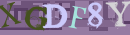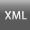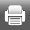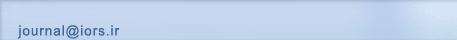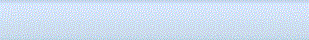[Home ] [Archive]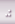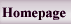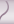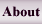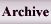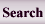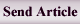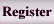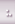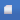Volume 2, Issue 1 (vol 2. No 1 2010)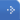IJOR 2010, 2(1): 41-49 Back to browse issues page
Linear plus fractional multiobjective programming problem with homogeneous constraints using fuzzy approach
Jain , Lachhwani
Abstract:   (23595 Views)

We develop an algorithm for the solution of multiobjective linear plus fractional programming problem (MOL+FPP) when some of the constraints are homogeneous in nature. Using homogeneous constraints, first we construct a transformation matrix T which transforms the given problem into another MOL+FPP with fewer constraints. Then, a relationship between these two problems, ensuring that the solution of the original problem can be recovered from the solution of the transformed problem, is established. We repeat this process of transformation until all the homogeneous constraints are removed. Then, we discuss the multi objective programming part, for which fuzzy programming methodology is proposed which works for the minimization of perpendicular distances between two hyper planes (curves) at the optimal points of the objective functions. A suitable membership function is defined with the help of the supremum perpendicular distance. A compromised optimal solution is obtained as a result of the minimization of the The supremum perpendicular distance. The corresponding optimal solution to the original problem is obtained using the transformation matrix. Finally, an example is given to illustrate the proposed model.

Keywords: Linear plus fractional functional, Transformation matrix, Distance function, Membership function.